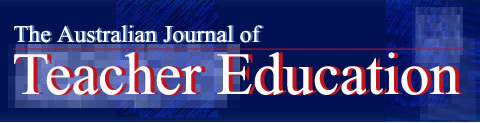•
•

## Australian Journal of Teacher Education### Effects of the Instruction with Mathematical Modeling on Pre-service Mathematics Teachers’ Mathematical Modeling Performance

#### Abstract

The purpose of the study was to investigate the effects of the instruction with mathematical modeling on pre-service mathematics teachers’ mathematical modeling performance. The participants were 24 pre-service elementary mathematics teachers. A mixed method approach was used to conduct the research. Each week, the participants were given two mathematical modeling problems and solved them as a group. After that, each group shared their solutions with the class so that there was an opportunity to focus on different solution methods. The data was collected via a pre and a post mathematical modeling test. SPSS package program was utilized in order to determine the significance level of the difference between the test results. One of the participants’ solutions in the pre-test and in the post-test was also analyzed to enhance the results. The result showed that the instruction based on mathematical modeling improved pre-service teachers’ mathematical modeling performance.

COinS

#### Link to publisher version (DOI)

10.14221/ajte.2018v43n8.1